# Activity Ratio Analysis – Accounts Class 12

Activity Ratio Analysis – Activity ratios are financial analysis tools used to measure a business’ ability to convert its assets into cash. These ratios are known as turnover ratios because they indicate the rapidity with which the resources available to the concern are being used to produce revenue from operations.

The different types of activity ratios are:

1.  Inventory Turnover Ratio
2. Trade Receivables Turnover Ratio
3. Trade Payables Turnover Ratio
4. Working Capital Turnover Ratio
5. Net Assets or Capital Employed Turnover Ratio

### 1. Inventory Turnover Ratio – Type of Activity Ratio

It indicates the relationship between the cost of revenue from operations during the year and average inventory during the year.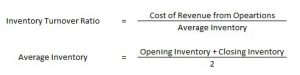Here, Cost of Revenue from Opearions = Opening Inventory + Purchases + Carriage Inward + Wages + Other Direct Charges – Closing Inventory

### 2. Trade Payables Turnover Ratio – Type of Activity Ratio

It indicates the relationship between credit purchases and average trade payables during the year.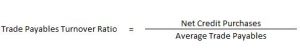Trade Payables include Creditors and Bills Payables.

### 3. Trade Receivables Turnover Ratio – Type of Activity Ratio

It indicates the relationship between credit revenue from operations and average trade receivables during the year.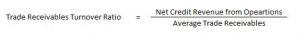### 4. Working Capital Turnover Ratio – Type of Activity Ratio

This ratio is important in non-manufacturing concerns where current assets play a major role in generating sales. It indicates the efficient use of working capital.

### 5. Net Assets or Capital Employed Turnover Ratio

It signifies the relationship that exists between sales of an enterprise and its net assets (capital employed). Higher the ratio the better it is, as it reflects the better performance of the business.

Formula for calculating the Net Assets or Capital Employed Turnover Ratio :

= Revenue from Operation/ Capital Employed

### Activity Ratio Examples

#### Calculation of current assets turnover ratio – Activity Ratio Analysis – Question 1

From the following information calculate the Current assets turnover ratio: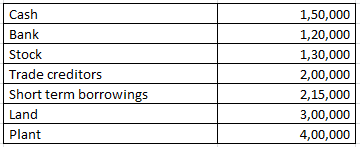Revenue from the operation for the year were RS. 2800000

Explanation : –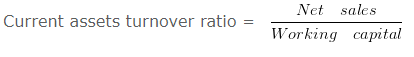Current assets turnover ratio = 2800000/400000

Current assets turnover ratio = 7 Times
Working note 1 : Current assets = Cash + Bank + Stock
Current assets = 150000 + 120000 + 130000
Current assets = 400000

#### Calculation of inventories when Inventory Ratio is given – Activity Ratio Analysis – Question 2

Calculate the value of opening Inventory from the following information.
Cost of revenue from operations is 1200000 and Inventory turnover ratio is 3 Times. and opening inventory is 40000 less than the closing inventory

Explanation : –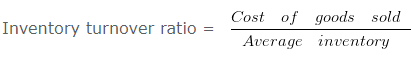3 = 1200000/Average inventory

Average inventory = 400000
Workings:

Opening inventory = 400000 (-)40000/2

Opening inventory 380000

Closing inventory = 400000 (+)40000/2

Closing inventory = 420000

#### Activity Ratio Analysis – Activity Ratio Analysis – Question 3

Calculate the value of opening Inventory from the following information:
Cost of revenue from operations is 16000 and Inventory turnover ratio is 1 Times. and opening inventory is 6 Times More than the closing inventory.

Explanation : –1 = 16000/Average inventory

Average inventory = 16000
Workings: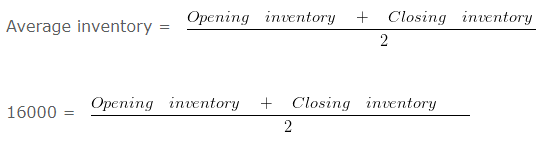32000 = Opening inventory + Closing inventory

Since the opening inventory is 6 Times More than the closing inventory therefore the ratio between opening inventory and closing inventory will be 7 : 1

Opening inventory = 32000 x 7/8

Opening inventory = 28000

Closing inventory = 32000 x 1/8

Closing inventory = 4000

#### When inventory and current assets given – Activity Ratio Analysis – Question 4

From the following information compute the current ratio.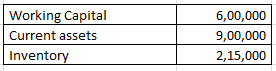Explanation : –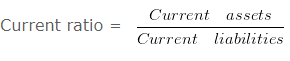Current Ratio = 900000/300000

Current Ratio = 3 :1
Workings:
Working Capital = Current assets (-) Current liabilities
600000 = 900000 (-) Current liabilities
Current liabilities = 300000

#### Fixed assets turnover ratio – Activity Ratio Analysis – Question 5

From the following information, calculate fixed assets turnover ratio:

Revenue from the operation for the year were RS. 2000000

Explanation : –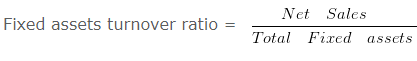Fixed assets turnover ratio = 2000000/500000

Fixed assets turnover ratio = 4 Times
Total Fixed assets = Land + Building + Furniture
Total Fixed assets = 300000 + 50000 + 150000
Total Fixed assets = 500000

#### Inventory turnover ratio and average age of inventory – Question 6

Calculate Inventory turnover ratio and average age of inventory from the following information: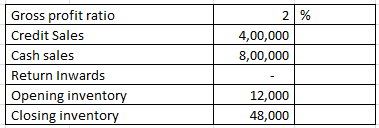Explanation : –Inventory turnover ratio = 1176000/30000

Inventory turnover ratio = 39.2 Times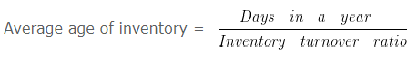Average age of inventory = 360/39.2

= 9.18 Days
Workings:
Cost of goods sold = Net Sales (-) gross profit
1200000 (-) 2% X 1200000
1200000 (-) 24000
1176000
Net sales = Cash sales + Credit Sales (-) Return Inwards
800000 + 400000 (-) 0
1200000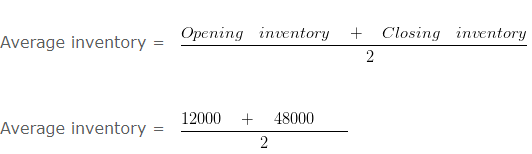= 30000

#### Inventory turnover ratio when closing stock is given – Activity Ratio Analysis – Question 7

Calculate Inventory turnover ratio from the following information: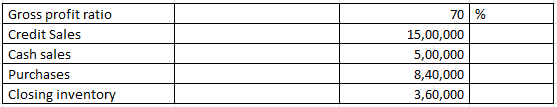Explanation : –Inventory turn over ratio = 600000/240000

= 2.5 Times
Workings:
Cost of goods sold = Net Sales (-) gross profit
= 2000000 (-) 70% x 2000000
= 2000000 (-) 1400000
= 600000
Net sales = Cash sales + Credit Sales
500000 + 1500000
2000000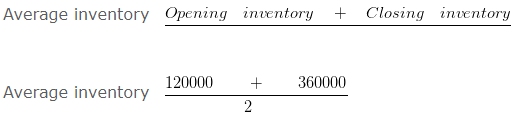Average inventory = 240000
Cost of goods sold = Opening inventory + Purchases (-) Closing inventory
600000 = Opening inventory + 840000 (-) 360000
= Opening inventory + 480000
Opening inventory = 120000

#### Inventory turnover ratio when GP ratio based on cost – Activity Ratio Analysis – Question 8

Calculate Inventory turn over ratio from the following information: Gross profit ratio is 10 % Of cost and revenue from operation is RS. 770000 . Opening inventory was 9/11 of closing inventory and closing inventory was 10 % of revenue from operation.

Explanation : –Inventory turnover ratio = 700000/70000

Inventory turnover ratio = 10 Times
Working note: = Gross profit ratio is 10% Of cost
Therefore goods costing Rs. = 100 is sold for RS. 110
Cost of goods sold = 100
If revenue from operation is = 770000

Cost of goods sold = 700000 ( 770000 X 100/110 )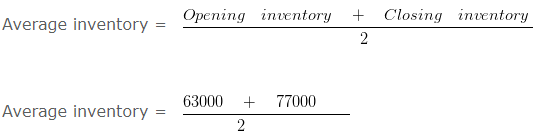= 70000
Closing inventory = 10% x Revenue from operation
Closing inventory = 10% x 770000
Closing inventory = 77000

Opening inventory = 9/11 x Closing inventory

Opening inventory = 9/11 x 77000

Opening inventory = 63000

#### Inventory turnover ratio when GP ratio is negative – Activity Ratio Analysis – Question 9

Calculate Inventory turnover ratio from the following information: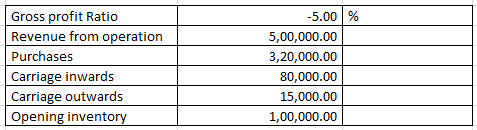Explanation : –Inventory turnover ratio = 525000/37500

Inventory turnover ratio = 14 Times
Workings:
Cost of goods sold = Revenue from operation (-) gross profit
= 500000 (-) -5% X 500000
= 500000 (-) -25000
= 525000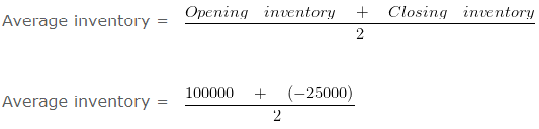= 37500

#### Inventory turnover ratio when opening stock is given – Activity Ratio Analysis – Question 10

Calculate Inventory turn over ratio from the following information: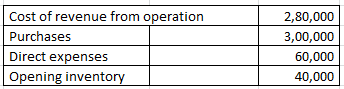Explanation : –Inventory turn over ratio = 280000/80000

Inventory turn over ratio = 3.5 Times
Workings:
Cost of goods sold = Opening inventory + Purchases + Direct expenses – Closing inventory
280000 = 40000 + 300000 + 60000 – Closing inventory
Closing inventory = 120000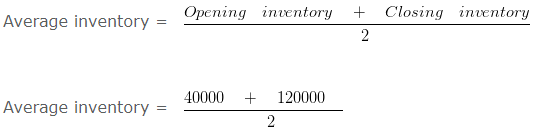Average inventory = 80000

#### Total assets turnover ratio – Question 11

From the following information calculate the total assets turnover ratio.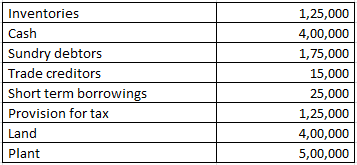Revenue from the operation for the year were RS. 4800000

Explanation : –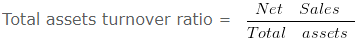Total assets turnover ratio = 4800000/1600000

Total assets turnover ratio = 3 Times
Working note 1 : Total assets = Inventories + Cash + Sundry debtors + Land + Plant
Total assets = 125000 + 400000 + 175000 + 400000 + 500000
Total assets = 1600000

#### Working capital turnover ratio – Question 12

From the following information calculate the working capital turnover ratio: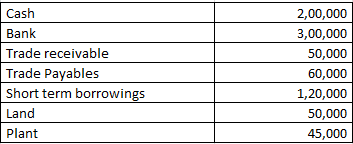Revenue from the operation for the year were RS. 1850000

Explanation : –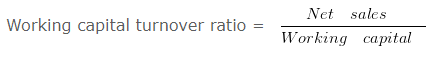Working capital turnover ratio = 1850000/370000

Working capital turnover ratio = 5 Times
Working note 1 : Working capital = Current assets (-) Current liabilities
Working capital = 550000 (-) 180000
Working capital = 370000
Working note 2 : Current assets = Cash + Bank + Trade receivable
Current assets = 200000 + 300000 + 50000
Current assets = 550000
Working note 3 : Current liabilities = Trade Payables + Short term borrowings
Current liabilities = 60000 + 120000
Current liabilities = 180000

#### Determination of current assets when current ratio is given – Question 13

Current Ratio of a business is 13 : 11 and Quick Ratio is 0.75 .
If Working Capital is Rs. 200000 then calculate the value of current assets and inventory.

Explanation : –

Working Capital = Current Assets (-) Current Liabilities
or
Current Liabilities = Current Assets (-) Working Capital
= 1300000 (-) 200000
= 1100000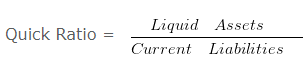or
Liquid Assets = Current Liabilities x Quick Ratio
= 1100000 x 0.75
= 825000
Inventory = Current Assets (-) Liquid Assets
= 1300000 (-) 825000
= 475000
Working Note 1 :
Working Capital = Current Assets (-) Current Liabilities
Working Capital = 13 (-) 11
Working Capital = 2
When working capital = 2 then Current Assets = 13
When working capital = 200000 then Current Assets = 1300000

#### Trade payable turnover ratio – Activity Ratio Analysis – Question 14

Calculate the trade payable turnover ratio and average payment period From the following information. Credit purchases during the 2016-2017 is 1000000 . Balance of opening Creditors and Bills payable on 01.04.2016 is RS. 40000 and 15000 and the Balance of Closing Creditors and bills payable on 31.03.2017 is RS. 35000 and 10000

Explanation : –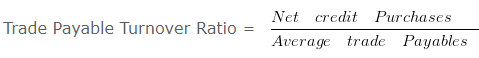Trade Payable Turnover Ratio = 1000000/50000

Trade Payable Turnover Ratio = 20 Times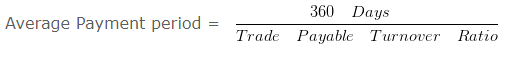Average Payment period = 360/20

Average Payment period = 18 Days
Workings: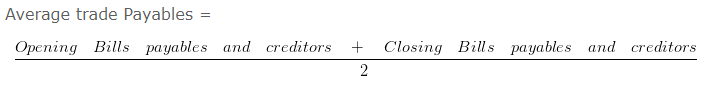Average trade Payables = 100000/2

Average trade Payables = 50000

#### Trade receivable turnover ratio – Activity Ratio Analysis – Question 15

Calculate the Trade receivable Turnover Ratio and average collection period from the following information: Total revenue from the operation is 100000 and cash revenue is 10 % of total revenue from the operation. Balance of opening receivable on 01.04.2016 is RS. 60000 and the Balance of Closing receivable on 31.03.2017 is 40000

Explanation : –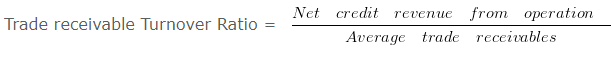Trade receivable Turnover Ratio = 90000/50000

Trade receivable Turnover Ratio = 1.8 Times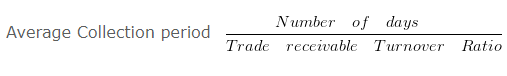Average Collection period = 360/1.8

Average Collection period = 200 Days
Workings:
Credit sales = 100000 x 0.9
= 90000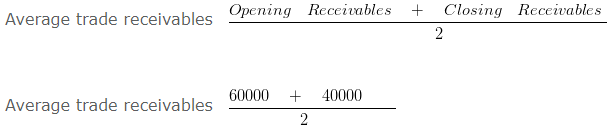Average trade receivables 50000